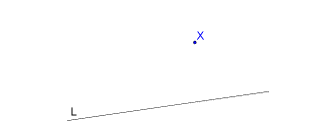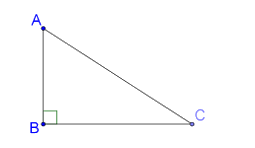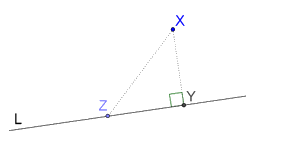# Distance of a Point From a Line

Distance of a Point From a Line
Go back to  'Triangles'

Suppose that you have a line L, and a point X which does not lie on L, as shown below:When we talk about the distance of X from the line L, what do we mean? How do we measure the distance of a point from a line?

To answer that question, first consider a triangle ABC, which is right-angled at B:Note that since ∠B = 90°, it is the largest angle in the triangle, which means that AC (the hypotenuse) is the largest side. This will always be true. The hypotenuse AC will always be larger than the perpendicular from A to BC, which is AB.

Returning to our point and line, let us drop a perpendicular from X onto L:Y is the foot of this perpendicular, while Z is any other different point on L. Note that XY will always be smaller than XZ, no matter where Z is on the line. In other words: the shortest distance of a point from a line is along the perpendicular dropped from the point onto that line.

Thus, the definition of the distance of a point X from a line L is: the length of the perpendicular dropped from X onto L.

grade 9 | Questions Set 2